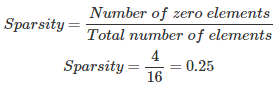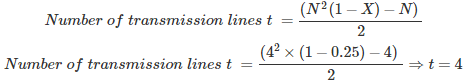# MCQs on Power Systems

##### Page 4 of 67. Go to page 1 2 3 4 5 6 7 8 9 10 11 12 13 14 15 16 17 18 19 20 21 22 23 24 25 26 27 28 29 30 31 32 33 34 35 36 37 38 39 40 41 42 43 44 45 46 47 48 49 50 51 52 53 54 55 56 57 58 59 60 61 62 63 64 65 66 67
01․ Advantages of shunt compensation is/are
single unit can be used as both capacitance and inductor by adjusting the excitation
smooth voltage regulation is possible by controlling excitation
It requires less maintanance
all of the above

Shunt compensation: 1. Two separate units are required, i.e. inductor and capacitor. 2. Step by step voltage regulation is possible 3. Cost of shunt compensation is low 4. It consumes only reactive power 5. It requires less maintenance because there is no running equipment 6. It does not requires starting mechanism

02․ Specified quantities of load bus are
P and Q
V and δ
P and δ
Q and V

Load bus is also known as PQ bus. Load bus is related to consumer requirements in which P and Q are known or specified quantities, for supplying these quantities δ and V are to be calculated. Load bus: Known quantities - P and Q Unknown quantities - V and δ Generator bus: Known quantities - P and V Unknown quantities - Q and δ Slack or reference bus: Known quantities - V and δ Unknown quantities - P and Q Where P = Active power, Q = Reactive power, V = voltage and δ = load angle.

03․ Specified quantities of slack bus are
P and Q
V and δ
P and δ
P and V

Every power system network containing one slack bus. This slack bus is used to supply active and reactive power requirements of the transmission line, for this purpose one of the generator bus which is most efficient is used as slack bus. Slack or reference bus: Known quantities - V and δ Unknown quantities - P and Q Where P = Active power, Q = Reactive power, V = voltage and δ = load angle.

04․ Which of the following quantities are to be calculated for generator bus?
P and V
P and Q
Q and δ
δ and V

In generator bus P and V are known quantities and range of Q is also specified for safe operation of equipments and Q and δ are top be calculated. Load bus: Known quantities - P and Q Unknown quantities - V and δ Generator bus: Known quantities - P and V Unknown quantities - Q and δ Slack or reference bus: Known quantities - V and δ Unknown quantities - P and Q

05․ In a 4*4 Y bus matrix the number of non zero elements are 12. Find the sparsity of the system?
0.25
0.5
0.75
1

Total number of elements in 4×4 Y bus matrix = 16 Number of zero elements = 406․ In a 4 bus,4×4 Y bus matrix the number of non zero elements are 12. Find the number of transmission lines?
8
4
2
5

N = Number of buses X = Sparsity Sparsity X = 4/16 = 0.2507․ Range of accelerating factor is
50 to 100
1 to 10
1.6 to 1.8
10.8 to 11.2

Accelerating factor is used for reducing number of iterations using gauss-siedel method. The range of accelerating factor is 1.6 to 1.8.

08․ A network containing 100 buses in which 10 are the voltage control buses, 5 are fixed shunt capacitor buses, 20 are the reactive power support buses, 6 are the generator buses. Find the size of the Jacobian matrix?
163 × 163
164 × 164
165 × 165
162 × 162

Size of the Jacobian matrix = (2n-m-2)×(2n-m-2) Where, n = Total number of buses m = Total number of PV buses m = Voltage control buses + Reactive power support buses + generator buses except slack bus Fixed shunt capacitors are supplying constant amount of reactive power, so that fixed shunt capacitors are considered as load buses or PQ buses. Therefore, n = 100 and m =35 Size of the Jacobian matrix = (2×100 - 35 -2)×(2×100 - 35 - 2) = 163×163

09․ Which of the following buses is/are not PV buses?
voltage control buses
fixed shunt capacitor buses
reactive power support buses
both 1 and 2

Size of the Jacobian matrix = (2n-m-2)×(2n-m-2) Where, n = Total number of buses m = Total number of PV buses m = Voltage control buses + Reactive power support buses + generator buses except slack bus Fixed shunt capacitors are supplying constant amount of reactive power, so that fixed shunt capacitors are considered as load buses or PQ buses.

10․ Which of the following is/are advantages of N-R method?
Number of iterations are less
Applicable for large power system network
Time taken for each iteration is less
both 1 and 2

Advantages of N-R method: 1. Number of iterations are less, so that it has fast convergence. 2. Convergence is not effected by the choice of slack bus. 3. No need of accelerating factor. 4. Applicable for large power system network. Disadvantages of N-R method: 1. Time taken for each iteration is larger if size of the Jacobian matrix is larger. 2. Computer memory required is larger. 3. Computer programming is difficult.

<<<23456>>>